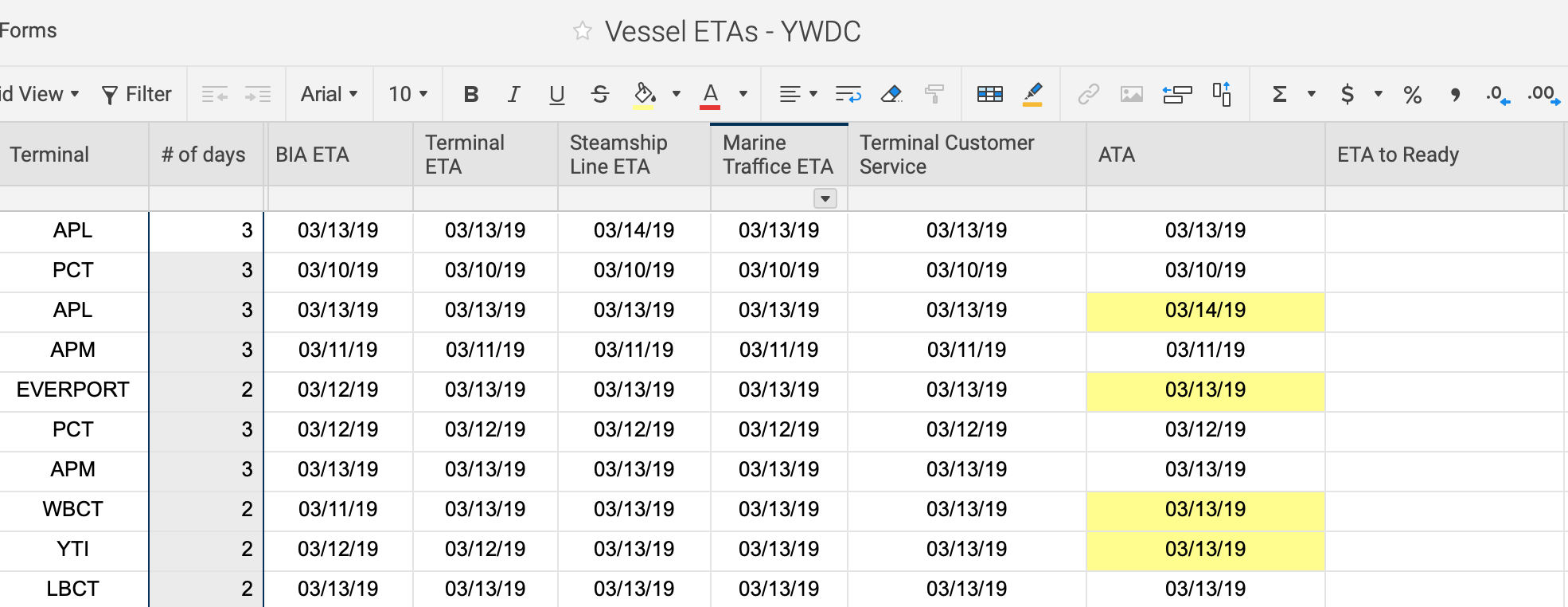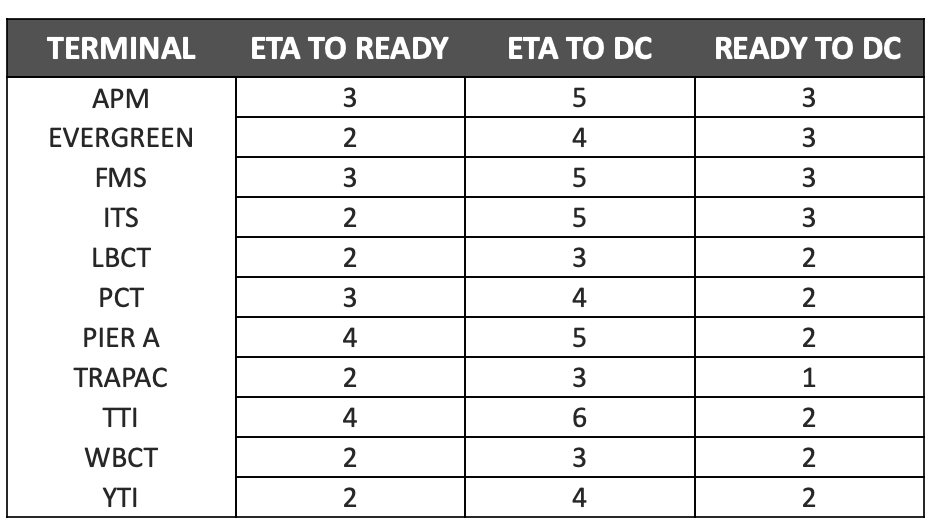# Needing Help with Multiple Logical Expressions Formula

I am working on a formula that will look at a few different cells and make a calculation.  I need the formula to look at

ETA to Ready = [terminal ETA] + [# of days]

The [# of days] is determined by which [Terminal]  So I need the formula to look at the value in the [Terminal] column, then add the associated [# of days] to the [Terminal ETA] date for the actual [ETA to Ready]

For example, if the Terminal ETA date is 4/10/19 and the Terminal is "APM" which is associated with a "3 day" I would want the formula to return a value of 4/13/19.  Not sure if I'm making sense with this formula, but would love some assistance.Tags:

• try this

= [terminal ETA]@row + [# of days]@row

• I don't think this will work.  The terminal value will vary and therefore that will drive the #of days calculation.  I need it to logically look at the terminal, then add the associated # of days with said terminal.

• are you saying this is your lookup table then? I don't really understand how the sheet is going to be used in that case. where is the result posted?

• My initial plan was to just get the if/then formula working by manually inputing the #ofdays column with the correct value for said terminal.  In the future I was going to develop a secondary formula in the #ofdays column to return the proper value depending on the value in the terminal column.  the end result needs to be in the "ETA Ready to Dray" column. Does this muddy the water or make it clear what I'm trying to do.  I appreciate you assistance.

• Is your [# of Days] column automated or manual entry?

thinkspi.com

• manual at the moment, but will be automated.

• [email protected]'s initial answer should work to give you your date.

Automating the [# of Days] column would be the step that makes or breaks efficiency.

I would suggest building out a table and using an INDEX/MATCH for that part.

If you are trying to completely do away with the [# of Days] column, then I still suggest building a table and using an INDEX/MATCH.

I guess the best question would be... Are you trying to keep or get rid of the [# of Days] column?

thinkspi.com

• yes ideally get rid of it.  See below for the table I'll be using.  I've never done an index match.  I will need to look into that more• And which column would be used to pull the number from for the date you are looking for? I would assume the first column?

Will this table be on the same sheet or a different sheet?

thinkspi.com

• It would be the second column (ETA to Ready) I will set it up as a reference sheet.

• =[Terminal ETA]@row + INDEX({Cross Sheet Reference ETA to Ready}, MATCH([email protected], {Cross Sheet Reference Terminal}, 0))

If you follow the appropriate steps for cross sheet referencing, simply select the column specified in the range in the formula above. This should do the trick for you.

thinkspi.com

• Thanks so much.  I was able to make this work. Thanks everyone!

• Excellent! Happy to help!thinkspi.com

## Help Article Resources

Want to practice working with formulas directly in Smartsheet?

Check out the Formula Handbook template!